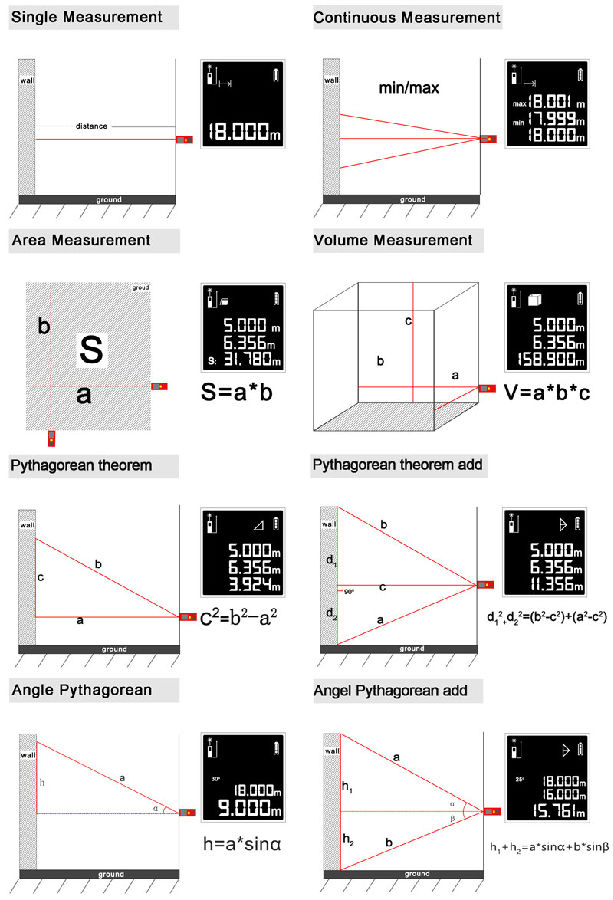# The principle and function of laser ranging

21-08-2019

Norm's laser range finder is a pulsed laser range finder. The pulsed laser range finder emits a beam or a series of short pulsed laser beams to the target during operation. The photoelectric component receives the laser beam reflected by the target. The timer measures the time from the launch of the laser beam to the reception and calculates the distance from the observer to the target.

What can the laser range finder measure? The following anwer will showing you.

1, distance distance is divided into several, the distance between the point and the point, the distance between the surface and the surface, etc., this is not introduced, there is an indirect measurement, for example, we measure the height of the house, if there is no direct contact between the roof and the ground We can use the indirect measurement method. The range finder itself calculates the distance of a specific position according to the mathematical Pythagorean theorem and the angle, making the measurement easier, as shown in the following figure:what about the rangefinder in the room measurement?

2, area, volume

The purpose of our measurement room is to measure the volume of the area. After the completion of the measurement room, we have to calculate it ourselves, and record the data. Now all of these can be realized by the rangefinder. The area volume is automatically calculated. The value is stored in the record.  Greatly convenient, Some of the range finder also have a side angle function, which makes the measurement more accurate.

Get the latest price? We'll respond as soon as possible(within 12 hours)